# The various components of the electric scheme

## Introduction

An electric scheme is a graphic representation of an electric circuit.

The various components of the electric scheme are:

• the components
• the wires or connections
• the junctions or the connecting points

the electric potentials (or equipotentials)

## Components

The components are chosen in a library of components.

From a graphic viewpoint, they are represented by the following symbols:

 Voltage/current sources Current source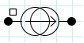Voltage source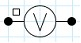Components of field-circuit coupling Coil conductor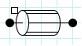Solid conductor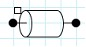Solid conductor N pins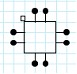R, L, C components Resistor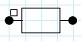Inductor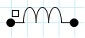Capacitor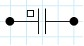Switch and Diode Switch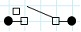Diode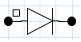Rotating machine components Brush-segment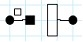Squirrel cage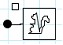Masse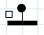## Connection

A connection is the representation of an electric wire.

From a graphic viewpoint, a connection is a sequence of segments and of changes of direction, between a starting point and an arrival point.

The authorized starting points and the arrival points are:

• the terminals of the component
• the extremities of the connection
• the angle of a change of direction of the connection
• the connecting points

## Connecting point

A connecting point is an intersection between wires with electric contact.

From a graphic viewpoint, a connecting point is represented by a point in bold.

## Electric potential

From a graphic viewpoint, an electric potential regroups the elements with the same potential (assembly of connections and of connecting points).

This is not about defining the quantity called electric potential.

## Example: electric scheme on sheet

Let us take the example of a simple electric circuit.

The circuit components are represented on the electric scheme on the drawing sheet (figures below).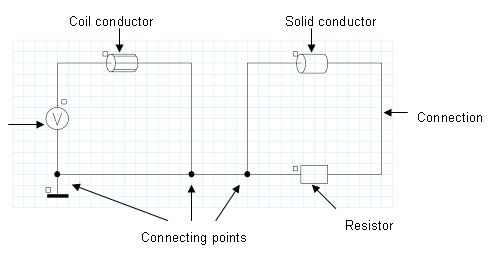## Example: representation of the tree of components

We are interested in the previous circuit example.

The circuit components are listed in the display zone corresponding to the components tree (figure below).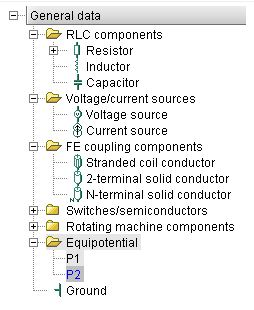The components tree comprises:

• the list of components
• a coil conductor B1
• a resistor R1
• a solid conductor M1
• a voltage source V1
• the list of potentials
• 3 equipotential entities, numbered as P1, P2, P3
• the Z1 ground point